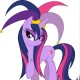Sky Slate Blueberry Blackcurrant Watermelon Strawberry Orange Banana Apple Emerald Chocolate# BGR to RGB issue.

5 replies to this topic• Members
• 786 posts
•Last active: Apr 10 2019 01:28 PM
• Joined: 17 Sep 2005
I wish I could remember who originally wrote this. Anyway I have been trying to figure out how to change BGR codes that you get from the windows color dialog to a format that Autohotkey GUI's can use correctly. So far the only thing I was able to find that actually worked correctly is.
```msgbox % rgb(255)
return
rgb(color){
SetFormat, integer, hex  ; Show RGB color extracted below in hex format.
Color := (Color & 0xff00) + ((Color & 0xff0000) >> 16) + ((Color & 0xff) << 16)
StringTrimLeft, Color, Color, 2
loop, % 6-strlen(Color)
Color=0%Color%
Color=0x%Color%
SetFormat, integer,D
return,color
}```
Is there another way to do this? Thanks in advance.• Members
• 1704 posts
•Last active: Nov 19 2015 11:58 AM
• Joined: 10 Mar 2011
i found this function which i use:

edit/ i guess you need to convert from decimal to hex too with this function

```msgbox, % DecToHex(FlipBlueAndRed(0xAABBCC))

;// http://www.autohotkey.com/forum/post-200921.html#200921
FlipBlueAndRed(c) ; takes RGB or BGR and swaps the R and B
{
return (c&255)<<16 | (c&65280) | (c>>16)
}

DecToHex(dec)
{
oldfrmt := A_FormatInteger
hex := dec
SetFormat, IntegerFast, hex
hex += 0
hex .= ""
SetFormat, IntegerFast, %oldfrmt%
return hex
}
```

i THINK yours might fail, if the each color isn't padded properly. but maybe not. i remember that was an issue with some other functions i found• Members
• 1177 posts
•Last active: Sep 11 2013 08:04 PM
• Joined: 22 Jun 2011
```#NoEnv

SendMode Input

SetWorkingDir %A_ScriptDir%

SetFormat integer , Hex

InputBox InitialValue

NewValue := ConvertColor( InitialValue )

MsgBox,

( LTrim

Initial Value: %InitialValue%

New Value: %NewValue%

)

return

ConvertColor( BGRValue )

{

BlueByte := ( BGRValue & 0xFF0000 ) >> 16

GreenByte := BGRValue & 0x00FF00

RedByte := ( BGRValue & 0x0000FF ) << 16

return RedByte | GreenByte | BlueByte

}```• Moderators
• 8510 posts
•Last active: Sep 10 2015 05:50 AM
• Joined: 08 Apr 2009
The script posted below has the function rgb_bgr_swap() This function will convert RGB <-> GBR

Using "SetFormat" is not needed.

The following is an explanation of the color value.
There are 3 components in the color "value" Red Green Blue
In the system that AutoHotkey uses, each of those components can have values in the range 0..255 (in hexadecimal thats 0x0..0xff)

Some don't understand how the values are combined into one value.
The answer is that the values are given different 'weight'.

Consider the number 123, it is the sum of 100 + 20 + 3.
In our decimal system the values for a digit may range 0..9
The total value is the sum of 1 group of 100, 2 groups of 20 and 3.
The weighting is by powers of 10: 1,10,100,1000 etc

In like manner, the color component are given similar weight.
In the color system each 'digit' may range 0..255
The weighting is by powers of 256: 1,256,65536
Each of the components R,G,B gets weighted by one of these values

In order to express the value R=1,G=2,B=3 using the RGB format,
use :arrow: (R * 65536) + (G * 256) + (To use BGR format, swap the weighing values for R & B.

There are actually 6 possible sequences for the letters RGB, fortunately on these two are used in AutoHotkey.

<!-- m -->http://www.autohotke... ... xpressions<!-- m -->

Integers and floating point:
...
Within expressions and non-expressions alike, integers may be written in either hexadecimal or decimal format.
Hexadecimal numbers all start with the prefix 0x. For example, Sleep 0xFF is equivalent to Sleep 255.

RGB & BGR color numbers are "sums" of the weighted R G & B components
Conversion between the formats involves swapping the R & B weighting

In the following script "n << 8" is the equivalent of "n * 2^8" or "n * 256"
In the following script "n >> 16" is the equivalent of "n / 2^16" or "n / 65536"

```#singleinstance force

loop, 15
a%a_index%:=1
red=11
green=22
blue=33

resultrgb:= rgbcolor(red,green,blue)
msgbox % resultrgb

resultbgr:= bgrcolor(red,green,blue)
msgbox % resultbgr

resultswap:= rgb_bgr_swap(resultrgb)
msgbox swapped %resultswap%

msgbox % resultrgb A_space resultbgr A_space resultswap

resultswap2:= rgb_bgr_swap(resultswap)
msgbox swapped %resultswap2%

rgbcolor(red=0,green=0,blue=0)
{
color:= (red << 16) + (green << 8) + blue
return color
}

bgrcolor(red=0,green=0,blue=0)
{
color:= (blue << 16) + (green << 8) + red
return color
}

; this swaps the color rgb <-> bgr
rgb_bgr_swap(color)
{
red:= ((Color & 0xff0000) >> 16)
green:= ((Color & 0x00ff00) >> 8)
blue:= (Color & 0xff)

color2:= (blue << 16) + (green << 8) + red
return color2
}

esc::exitapp```• Members
• 1177 posts
•Last active: Sep 11 2013 08:04 PM
• Joined: 22 Jun 2011

Using "SetFormat" is not needed.

You are correct that it is not needed for the ConvertColor function. It is needed, however, so that the MsgBox will display the input and output values in hex. Displaying those values in hex makes it easier to see that the high and low words are swapped.• Moderators
• 8510 posts
•Last active: Sep 10 2015 05:50 AM
• Joined: 08 Apr 2009

Displaying those values in hex makes it easier to see that the high and low words are swapped.

That is definitely true.